Civil Engineering - Steel Structure Design - Discussion

Discussion :: Steel Structure Design - Section 2 (Q.No.28)

28.

If p and d are pitch and gross diameter of rivets, the efficiency (η) of the riveted joint, is given by

 [A].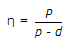[B].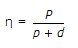[C].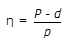[D].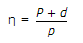[E].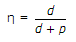Explanation:

No answer description available for this question.

 Raj said: (Dec 8, 2014) Is that answer correct? Is it mean when gross diameter of rivet zero there will be 100% efficiency?

 Vikas said: (Sep 18, 2017) @ Raj, As gross diameter is zero means there is no rivet in the plate, So there is 100% efficiency.

 Hardik said: (Jan 29, 2020) According to me; Efficiency(n) = 0.6d. Because n =(2.5d-d)/2.5d. = 1.5d/2.5d. = 0.6d.